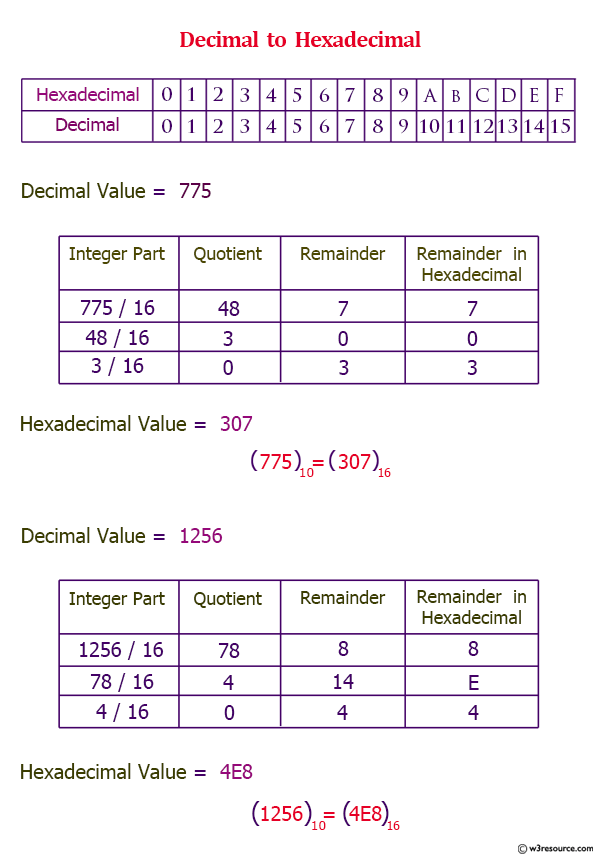# Python: hex() function

## hex() function

The hex() function converts an integer number to a lowercase hexadecimal string prefixed with "0x".

Version:

(Python 3.2.5)

Syntax:

```hex(x)
```

Parameter:

Name Description Required /
Optional
x An integer number Required

Example: Python hex() function

``````number = 127
print(number, 'in hex =', hex(number))

number = 0
print(number, 'in hex =', hex(number))

number = -35
print(number, 'in hex =', hex(number))

returnType = type(hex(number))
print('Return type from hex() is', returnType)
``````

Output:

```127 in hex = 0x7f
0 in hex = 0x0
-35 in hex = -0x23
Return type from hex() is <class 'str'>
```

Example: hex() function, representation of a float

``````number = 5.25
print(number, 'in hex =', float.hex(number))

number = 0.0
print(number, 'in hex =', float.hex(number))

number = 15.5
print(number, 'in hex =', float.hex(number))
``````

Output:

```5.25 in hex = 0x1.5000000000000p+2
0.0 in hex = 0x0.0p+0
15.5 in hex = 0x1.f000000000000p+3
```

Pictorial Presentation:Python Code Editor:

Previous: help()
Next: id()

Test your Python skills with w3resource's quiz

﻿

## Python: Tips of the Day

How do I check if a list is empty?

For example, if passed the following:

```a = []
if not a:
print("List is empty")
```

Ref: https://bit.ly/2A4JXx9